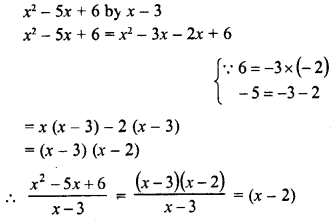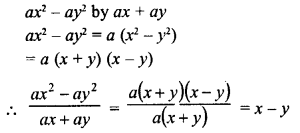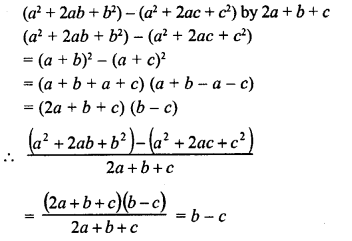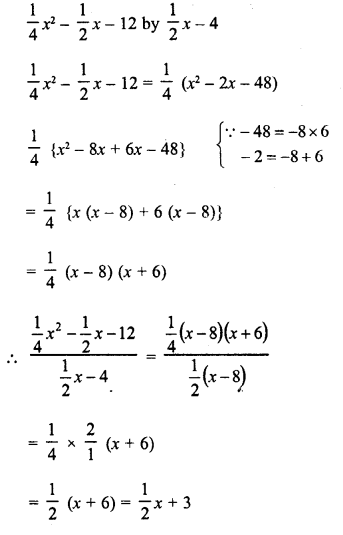## RD Sharma Class 8 Solutions Chapter 8 Division of Algebraic Expressions Ex 8.6

These Solutions are part of RD Sharma Class 8 Solutions. Here we have given RD Sharma Class 8 Solutions Chapter 8 Division of Algebraic Expressions Ex 8.6

Other Exercises

Divide :

Question 1.
x2 – 5x + 6 by x – 3
Solution:Question 2.
ax2 – ay2 by ax + ay
Solution:Question 3.
x– y4 by x– y2
Solution:Question 4.
acx2 + (bc + ad)x + bd by (ax + b)
Solution:Question 5.
(a2 + 2ab + b2)- (a2 + 2ac + c2) by 2a + b + c
Solution:Question 6.
$$\frac { 1 }{ 4 }$$ x– $$\frac { 1 }{ 2 }$$ x- 12 by $$\frac { 1 }{ 2 }$$ x – 4
Solution:Hope given RD Sharma Class 8 Solutions Chapter 8 Division of Algebraic Expressions Ex 8.6 are helpful to complete your math homework.

If you have any doubts, please comment below. Learn Insta try to provide online math tutoring for you.